# SAT II Math II : Ratios and Proportions

## Example Questions

### Example Question #71 : Mathematical Relationships

Solve the proportion: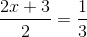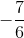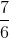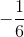Explanation:

To solve the proportion, cross multiply the terms.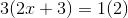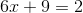Subtract 9 on both sides.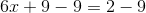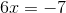Divide by 6 on both sides.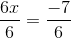The answer is:### Example Question #72 : Mathematical Relationships

Solve the ratio: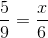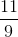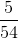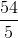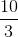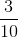Explanation:

To solve the proportion, cross multiply.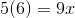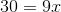Divide by 9 on both sides.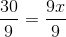Reduce the fractions.

The answer is:### Example Question #73 : Mathematical Relationships

Determine the value of: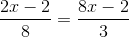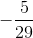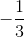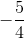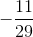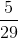Explanation:

Cross multiply the fractions.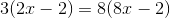Simplify both sides.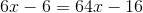Subtract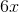on both sides.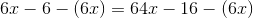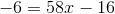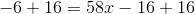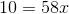Divide by 58 on both sides.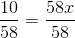Reduce both fractions.

The answer is: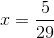### Example Question #74 : Mathematical Relationships

Solve the proportion: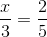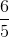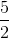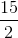Explanation:

Cross multiply both sides.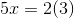Simplify and solve for x.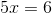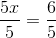The answer is:### Example Question #75 : Mathematical Relationships

Solve the proportion: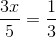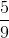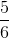Explanation:

Cross multiply both sides.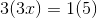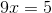Divide by 9 on both sides.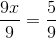The answer is:### Example Question #76 : Mathematical Relationships

Solve the proportion: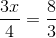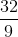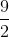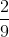Explanation:

Cross multiply the two fractions.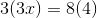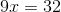Divide by nine on both sides.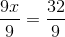The answer is:### Example Question #77 : Mathematical Relationships

Solve the proportion: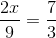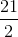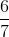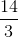Explanation:

Cross multiply the two fractions.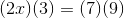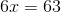Divide by six on both sides.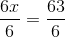The answer is: